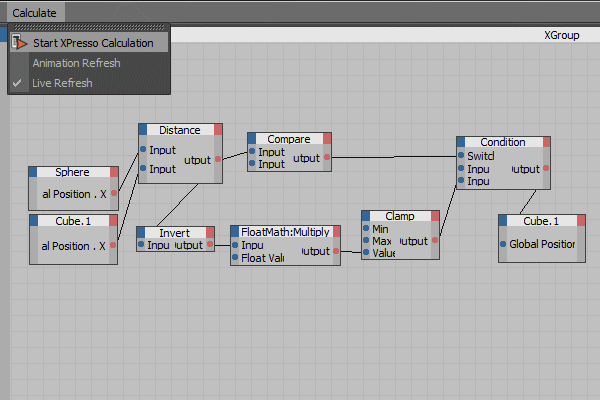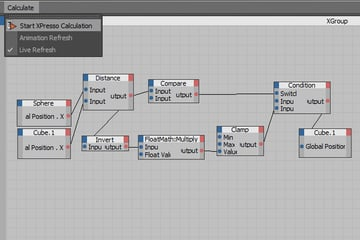# Creating An Autonomous Door System With Xpresso in Cinema 4D

In this tutorial you'll be learning how to create an autonomous door system using the Xpresso system in Cinema 4D. This introductory tutorial will teach you the basics of the Xpresso system and the application of it's own modules, and thus enable you to adapt that knowledge to any other creative idea.

### Final Effect Preview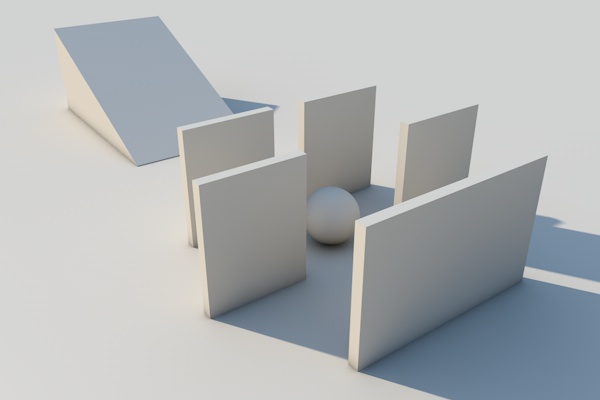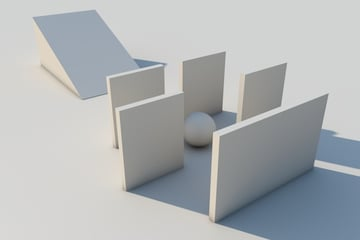### Step 1

Let's start by creating a Floor, and a Sphere. These are very basic shapes which you can find under the menus. I suggest you to use the "floor object" rather than creating a "plane object", since the floor object provides you an infinitely long surface.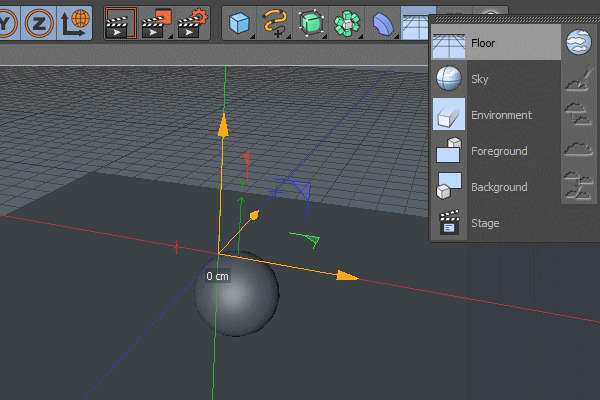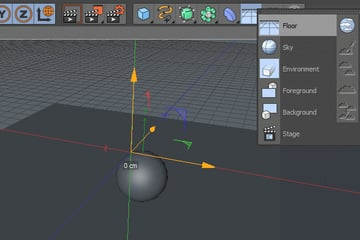### Step 2

Now create an inclined surface. Think simple, just create a new Box object and then make it editable (shortcut: C key) and lower one of the edges to get a ramp.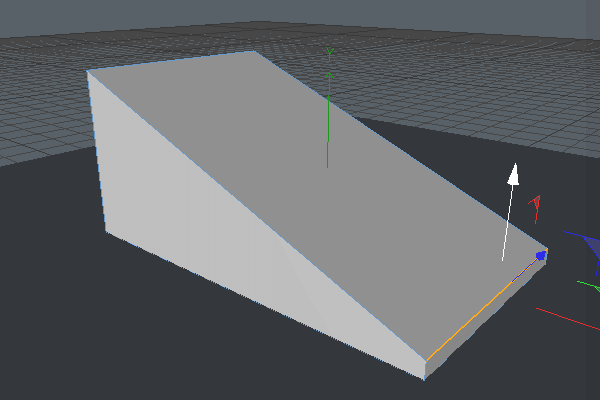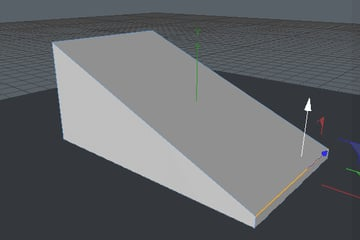### Step 3

Now assign the "Rigid Body" tag to all three objects: Floor, Sphere and Ramp.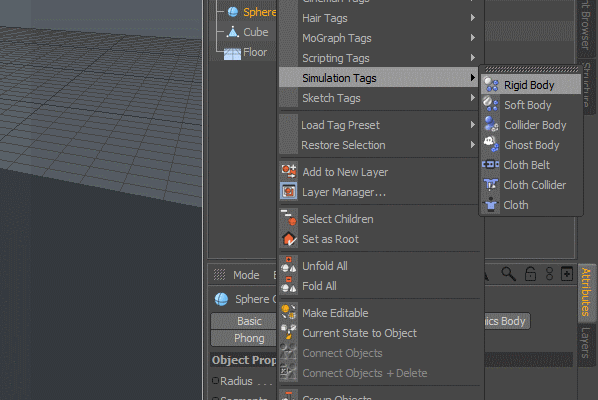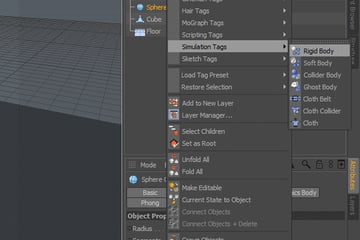### Step 4

Since the floor and ramp act like stationary objects, you have to turn off their dynamics. To do that, click on the "Rigid body" tag you assigned before and change the aforementioned setting to "Off".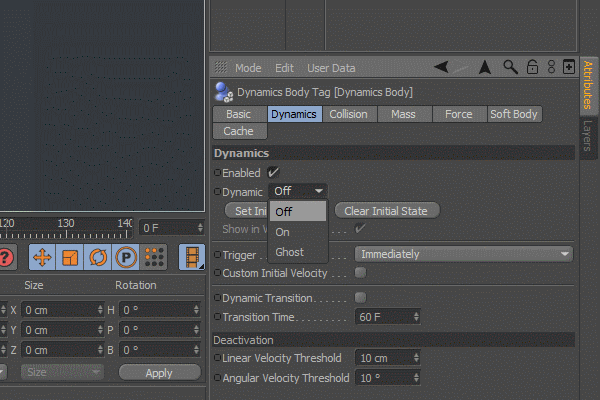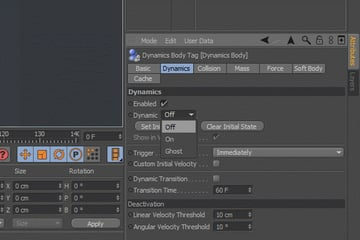### Step 5

Now create a "Null object" and then assign a "Xpresso tag" to it.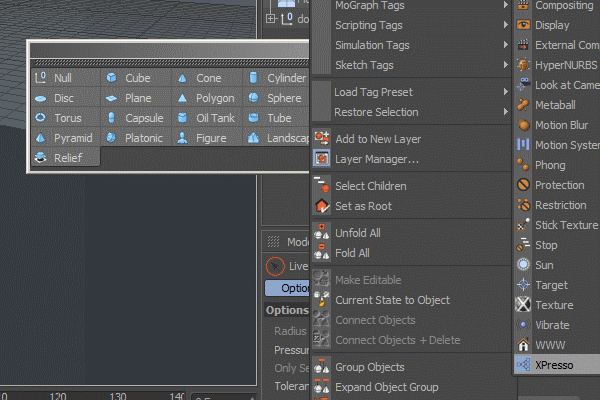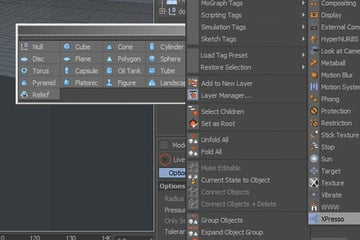### Step 6

Before going into Xpresso, we have to create the doors which we'll be controlling. So create a new Box object and shape it as you wish. Now by using a Symmetry object, duplicate the door. So when you move one side of the door, let's say to right. The other side will be moving to the left.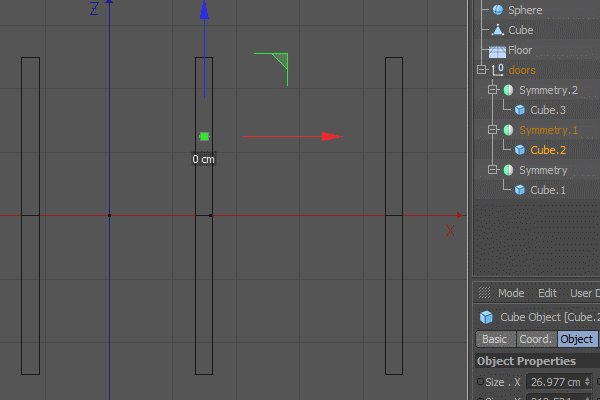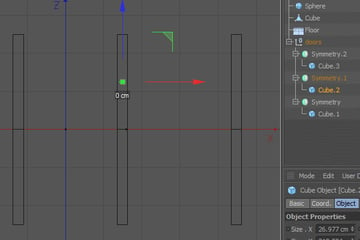### Step 7

Now double click on the Xpresso tag you assigned before. You'll see an Xpresso editor window. In the left column, there are two tabs X-Manager and X-Pool Jump to X-Pool, under the "system operators" you'll see "Xpresso", that's what we'll be using throughout the tutorial. All the modules are listed under the appropriate categories.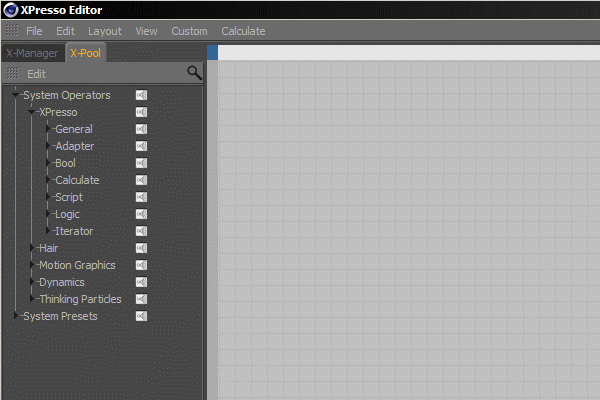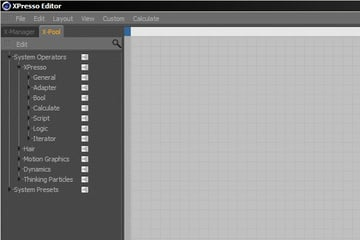### Step 8

In this Xpresso system the ball and doors will interact in a way. So we have to get them into the Xpresso editor. To do that, just simply drag and drop the Sphere object, and for now, one of the doors (But just the cube object, not the whole door with the symmetry object.)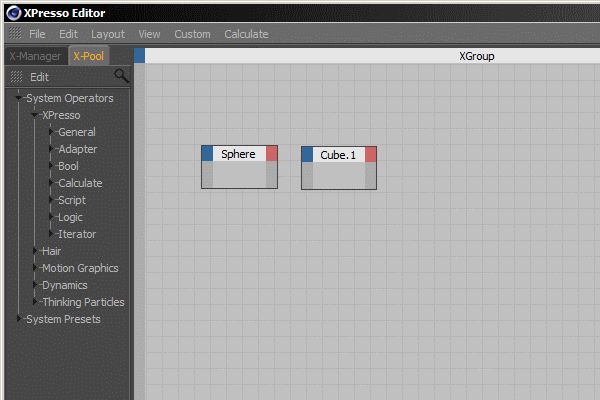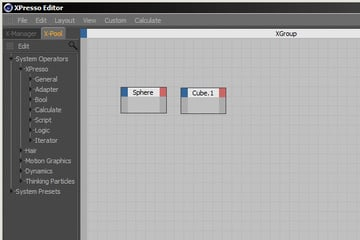### Step 9

As the ball object gets closer to door, the door will slowly open. To do that, we first have to calculate the distance between these two objects. We'll simply use the "Distance" module under the "Calculate" category. So just find it and drag and drop it to the work area.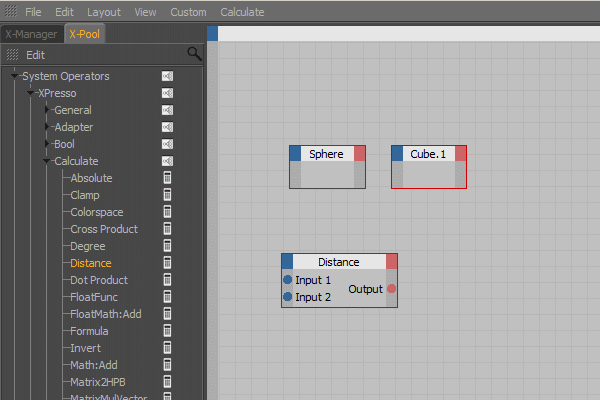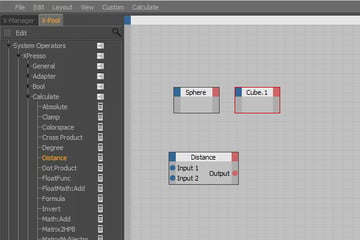### Step 10

Now there are two inputs and one output on the "Distance" module. What we have to do is providing two inputs. These will be the position of the sphere object and the door. To get that bunch of information from both the sphere and door, we have to create an output on them.

To do that simply click on the red corner of the sphere object and enable "global position x". How to decide which coordinate you have to use for the global position (x, y or z) depends on the coordinate pivot in the viewfinder. At the left bottom there is a little pivot which helps you.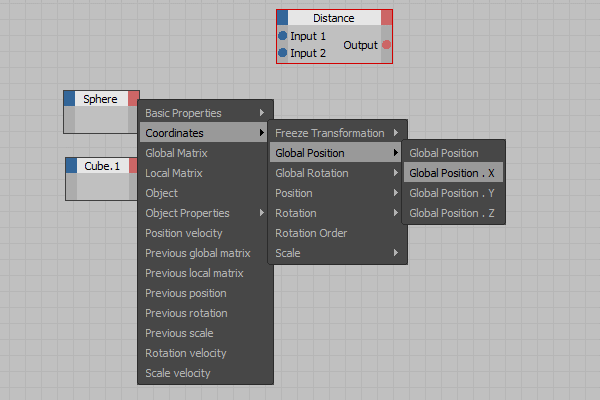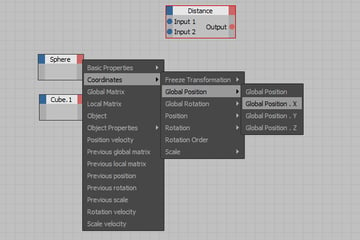### Step 11

So we have the two outputs from the objects, now we have to channel that information to the distance module for calculation. Simply click on the red dot and drag while holding the mouse button until you come over the blue input dot, that's pretty intuitive.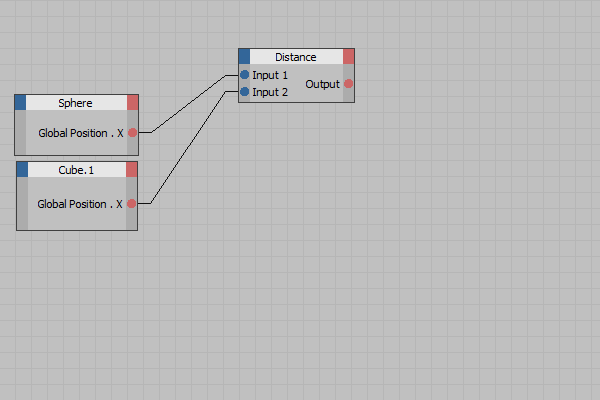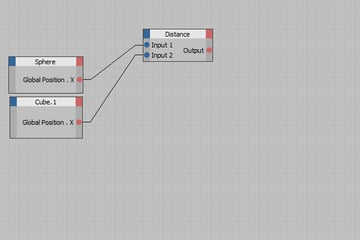### Step 12

The motion of the door will be triggered in accordance with the distance between the sphere and door. So, we have to determine a certain distance and whenever the ball goes beyond that distance, the door will be start to open. The closer the ball gets, the further the door opens. To do that we have to use the "compare" module as a trigger. Again we have two inputs. In this example one of the inputs will be coming from the distance measurement and the other input will be predetermined by the us.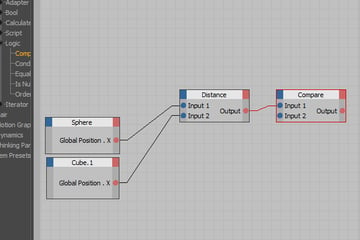### Step 13

Now click on the "Compare" module and jump to the Node tab under the Attributes menu. There is an option called "Function". This one determines the rules of comparison. Since we want it to get triggered when a distance is violated, we have to use " <= " or " < " .

The first one stands for "Less or equal" and second one stands for "Less". Choose any one you wish, it doesn't make a huge difference. And under the "Parameter" tab, there is "Input2" which will be predetermined. So, this will be the distance between the sphere and door before the "compare" module triggered. It depends on your animation scene, I used "800" but you can change it anytime you want, so just assign something around 800.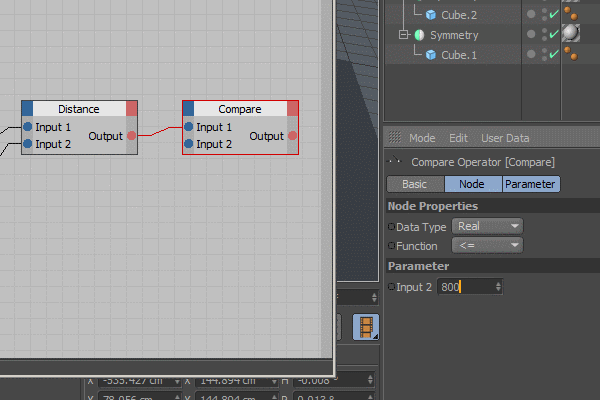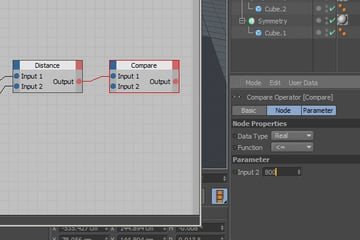### Step 14

Get the "Condition" module from the "logic" category. This one has three inputs; "Switch" is for triggering that module. If you input it with "1" (which stands for "true" in logic), it will change from "Input1" to "Input2". So we have to connect the output of the "Compare" module to the "Switch" input of the "Condition" module, thus we can trigger this module whenever the predetermined distance between the sphere and door is violated. Because when this distance is violated, the "Compare" module will be outputting "1", which means "true".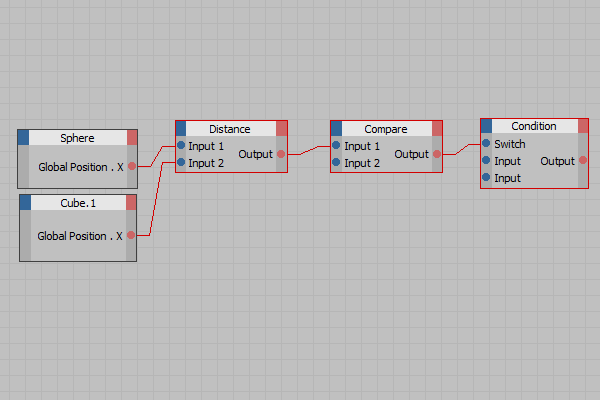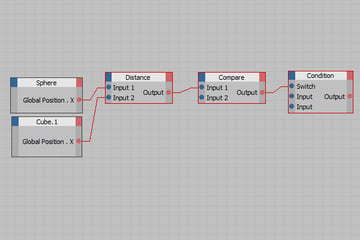### Step 15

So far so good, now we have to determine the values for "Input1" and "Input2" on the "Condition" module. These values correspond to the position of the door on the "Z-axis" (here it is the "Z-axis on my scene, but as I mentioned before, it depends on how you designed your scene, so you have to check the directions before determining on which axis you want to move your door) So the first input must be the initial position of the door on the Z-axis. Here it is "124.935" for me. (Also the axis pivot I've mentioned is visible on the screen shot, the green, blue and red pivot on the left side.) Use that value for first the input under the parameter tab.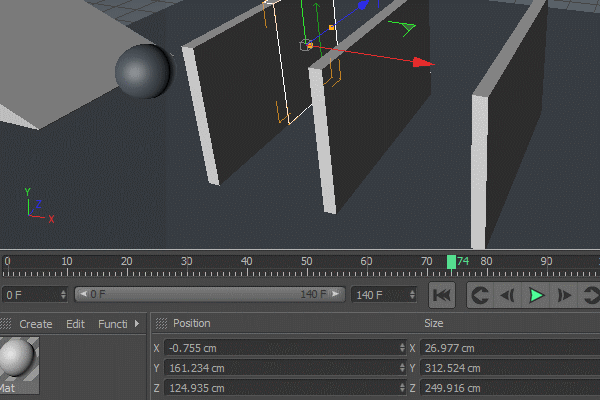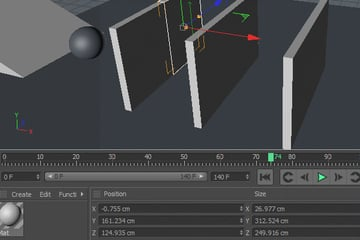### Step 16

Now the tricky part is determining the second input which will be changing as the ball gets closer to the door. So this value must be coming from the distance between the ball and the door. However, what we need is an increasing value. As we have a decreasing value between the ball and door, somehow we have to convert it.

Here the module we have to use is the "Invert" module. This module converts the high values to low values. So as it's input increases, the output decreases. Get it from the "Calculate" category and then connect the output of the "Distance" module to input of the "Invert" module.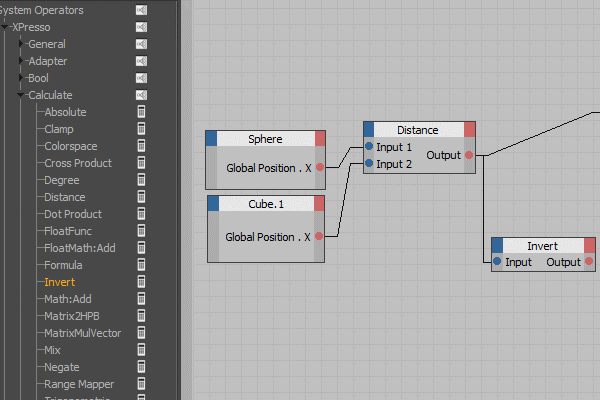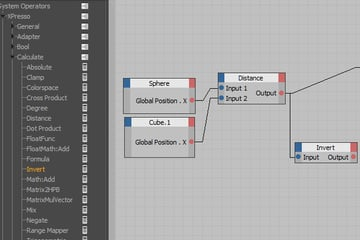### Step 17

If you connect a "result" module to the output of the "Invert" module, you'll see that the value you get is extremely low. So, somehow you have to magnify it. To do that, under the "calculate" category you have to use the "FloatMath:Add" module.

At first it looks like the addition operator, but you can change its function and use it as a multiplier. Turn it into a multiplier and then connect the output of "Invert" to the input of the "FloatMath" module. Then as a "Float value" enter a value around "50000". Again you can tweak it to whenever you want. This value is the number you multiply the input you get from the "Invert" module. The output of the "Invert" module is too low that multiplication with a big number is necessary to utilize it.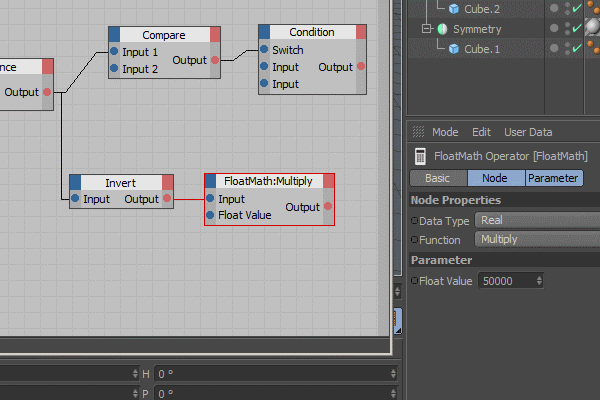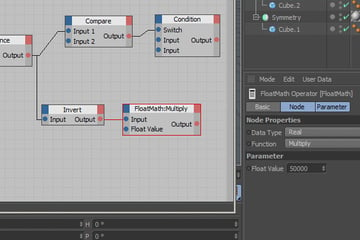### Step 18

Now we will face another problem that the output of the "FloatMath" module will reach high values to such a degree that the door will move too far away. So we have to clamp that output and determine the limits. In order to do that add the "Clamp" module, which is under the "Calculate" category" and then connect the output of "FloatMath" to the "Value" part of the "Clamp" module.

Now we have to determine the limits which will be clamped. The Min. value must be the initial "Z-axis" position of the door. That was "124.935" and the Max. value is the final position of the door on the Z-axis (I used "300" for the max.)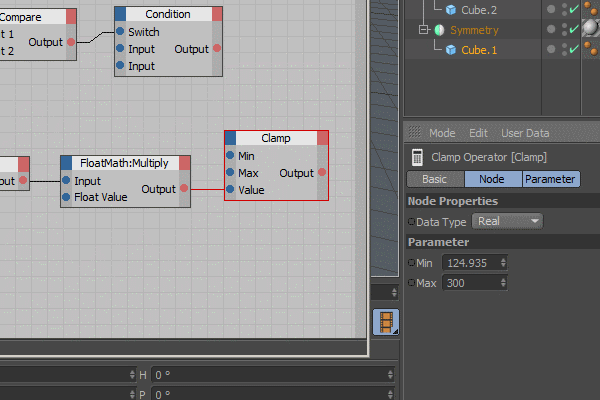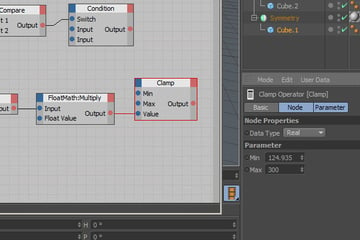### Step 19

The Output of the "Clamp" module will be the second input of the "Condition" module, which is the changing value from 124.935 to 300.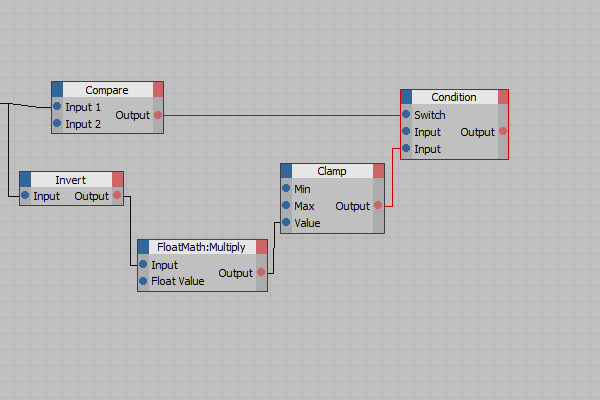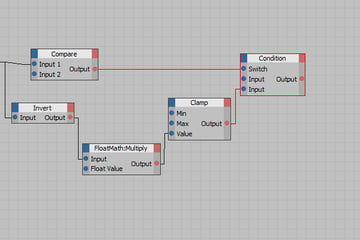### Step 20

Now at the end we have a value raising from 124.935 to 300 when the ball gets closer than 800cm to the door object. Now we have to use that output as an input on the door's "global position.z" To do that just drag and drop the door object (again just the cube object in the Symmetry object, not the whole door) and this time click on the left blue corner to add "Global Position. Z". And then connect the output of "Condition" to that new input of the door object (which is called "cube.1" on the screenshot.)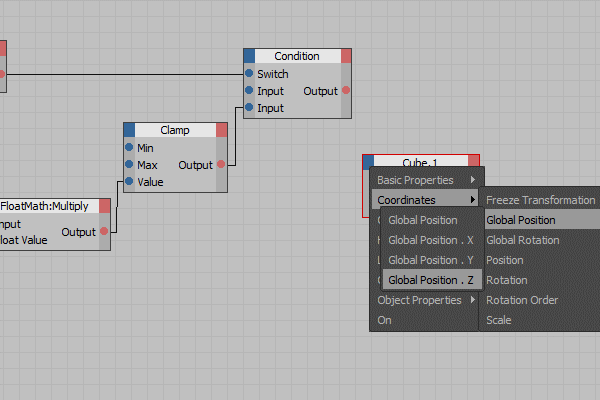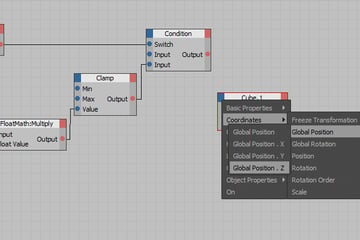### Step 21

The final look of the workspace is below. To initialize the Xpresso system, you have to start the Xpresso calculation. Which is also shown on the screenshot. So far I've explained how to control one of the doors. So, simply just duplicate the whole node system and use the new door (cube) objects to control the other two doors.